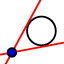# IM2.7 T9: A special inscribed (?) angle

Author:
00JonLind

1. Construct a circle with center A through point B. 2. Construct point C (≠B) on the circumference of the circle. 3. Construct chord BC so that BC is NOT a diameter. 4. Construct a tangent linethrough point B. 5. You should have a big arc and a small arc on your circle now. On the small arc, construct point D, segments BD and CD, and angle BDC. 6. Construct point E on the tangent line on the side of the big arc, and use it to measure angle CBE.

1. What kind of angle is BDC? 2. Move point D, and describe what happens to angle BDC. Why do you think this is true? 3. What is the relationship between angles BDC and DBE? Why do you think this is true? (Hint: what happens when you slide D close to B?) 4. Summarize what you've learned in your best academic language. What is the relationship between an angle formed by a chord and a tangent, and the arc it opens onto?

Find angles a, b, and c.